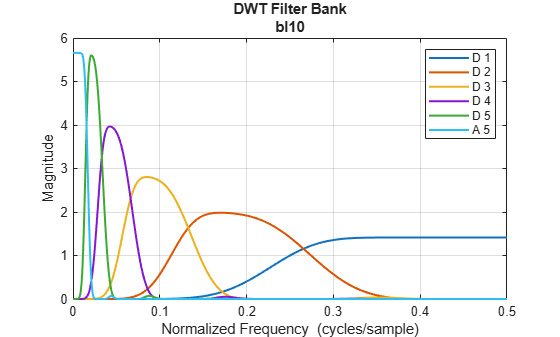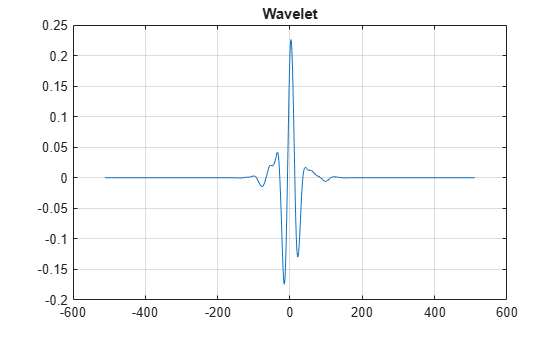# blscalf

Best-localized Daubechies scaling filter

Since R2022b

## Syntax

``scalf = blscalf(wname)``

## Description

example

````scalf = blscalf(wname)` returns the best-localized Daubechies scaling filter corresponding to `wname`.```

## Examples

collapse all

Obtain the scaling filter corresponding to the best-localized Daubechies wavelet with 10 vanishing moments. Confirm the sum of the filter coefficients nearly equals $\sqrt{2}$ and the L2 norm of the filter nearly equals 1.

```scalf = blscalf("bl10"); sum(scalf)-sqrt(2)```
```ans = -2.2204e-16 ```
`norm(scalf,2)`
```ans = 1.0000 ```

Use `orthfilt` to obtain the scaling and wavelet filters corresponding to the wavelet.

`[LoD,HiD,LoR,HiR] = orthfilt(scalf);`

Confirm the filters form an orthonormal perfect reconstruction wavelet filter bank.

`[tf,checks] = isorthwfb(LoD)`
```tf = logical 1 ```
```checks=7×3 table Pass-Fail Maximum Error Test Tolerance _________ _____________ ______________ Equal-length filters pass 0 0 Even-length filters pass 0 0 Unit-norm filters pass 1.7665e-10 1.4901e-08 Filter sums pass 7.2923e-15 1.4901e-08 Even and odd downsampled sums pass 3.7748e-15 1.4901e-08 Zero autocorrelation at even lags pass 7.3088e-11 1.4901e-08 Zero crosscorrelation at even lags pass 1.3089e-17 1.4901e-08 ```

Create a discrete wavelet transform filter bank using the wavelet. Plot the frequency responses of the wavelet filters and the final resolution scaling filter for the default signal length.

```fb = dwtfilterbank(Wavelet="bl10"); freqz(fb)```Plot the wavelet at the coarsest scale.

```[psi,t] = wavelets(fb); plot(t,psi(end,:)) grid on title("Wavelet")```Plot the scaling function at the coarsest scale.

```[phi,t] = scalingfunctions(fb); plot(t,phi(end,:)) grid on title("Scaling Function")```## Input Arguments

collapse all

Best-localized Daubechies wavelet, specified as one of these:

• `"bl7"` — Best-localized Daubechies wavelet with seven vanishing moments

• `"bl9"` — Best-localized Daubechies wavelet with nine vanishing moments

• `"bl10"` — Best-localized Daubechies wavelet with 10 vanishing moments

## Output Arguments

collapse all

Scaling filter corresponding to `wname`, returned as a vector. `scalf` should be used in conjunction with `orthfilt` to obtain scaling and wavelet filters with the proper normalization. The scaling filters agree exactly with Doroslovački . The sum of filter coefficients is nearly √2 and the L2 norm is nearly 1.0.

Data Types: `double`

 Doroslovački, M.L. “On the Least Asymmetric Wavelets.” IEEE Transactions on Signal Processing 46, no. 4 (April 1998): 1125–30. https://doi.org/10.1109/78.668562.